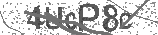﻿ How to Divide Fractions | Safe Videos for Kids
Welcome

# How to Divide Fractions

Thanks! Share it with your friends!

URL

You disliked this video. Thanks for the feedback!

Sorry, only registred users can create playlists.URLFind Related Videos  added
322 Views## Description

Watch more Basic Math Skills videos: http://www.howcast.com/videos/262765-How-to-Divide-Fractions

Math can be scary, but don't give up. Follow these simple steps, and soon you'll be dividing fractions like a pro.

Step 1: Write the problem
Write out the first fraction, a divided-by sign, and the second fraction. This will help you visualize the steps you need to take to divide the fractions.

Step 2: Invert the second fraction
Invert the second fraction by flipping it so that the bottom number is at the top. Rewrite the problem with the first fraction multiplied by the second, now inverted, fraction.

Tip
For example, rewrite one-half divided by three-fourths as one-half multiplied by four-thirds.

Step 3: Multiply the top two numbers
Multiply the top two numbers in the fractions to get the top number in your answer, which is known as the numerator.

Step 4: Multiply the bottom two numbers
Multiple the bottom two numbers in the fractions to get the bottom number in the answer, which is known as the denominator.

Tip
The result in the example is four-sixths.

Step 5: Reduce the final answer
Reduce the answer by dividing both the numerator and the denominator by the greatest common factor, which is the highest number by which both can be divided.

Tip
In the example, divide the numerator and denominator by two, the greatest common factor. The final answer is two-thirds.

Add an equal sign and your final, reduced answer at the end of the original problem. Keep practicing, and soon you may be solving tough math puzzles without even needing a pencil and paper.

Did You Know?
The word fraction comes from the Latin frangere, which means, "to break."Be the first to comment